## Chemistry Aptitude Quiz

IIT JEE exam which consists of JEE Main and JEE Advanced is one of the most important entrance exams for engineering aspirants. The exam is held for candidates who are aspiring to pursue a career in the field of engineering and technical studies. Chemistry is important because everything you do is chemistry! Even your body is made of chemicals. Chemical reactions occur when you breathe, eat, or just sit there reading. All matter is made of chemicals, so the importance of chemistry is that it's the study of everything..

Q1. Beta-emission takes place
•  From the elements above the band of stability
•  When neutron is converted to proton
•  With shifting of the new element one group towards right
•   Following all the facts given above
Solution
Part D

Q2.The angular momentum of an electron in 4s orbital, 3p orbital, and 4th orbit are
•  0,1/√2,h/π,2h/π
•  1/√2,h/2,2h/π,0
•  0,(√2 h)/π,4h/π
•  (√2 h)/π,4h/π,0
Solution
(a)
2K, 8L, 9M, and 2N 1s^2 2s^2 2p^6 3s^2 3p^6 3d^1 4s^2 (■(K means n=1,L means n=2 @M means n=3,N means n=4 )) Structure is 3d^1,4s^2
Atomic number 21
The total number of pe^-
2p^6+3p^6=12

Q3.   Slow neutrons can bring about the fission of
•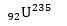•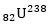••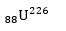Solution
Part A
Q4.   When passing through a magnetic field the largest deflection is experienced by
•  α-rays
•  β-rays
•  γ-rays
•  All equal
Solution
Part B

Q5.•  After two hours, less than 10% of the initial X is left
•  Maximum amount of Y present at any time before 30 min is less then 50% of the initial amount of X
•  Atomic numbers of X and Z are same
•  All of the above are correct statements
SolutionQ6. 4 Be^7captures a K electron into its nucleus. What is the mass number and atomic number of the nuclide formed?
•  3,7
•  4,8
• 3,8
•  4,7
Solution
(a)
4Be^7+ (-1) e^0 → 3 Li^7 So, atomic number=3, mass number=7

Q7.When electronic transition occurs from higher energy state to lower energy state with energy difference equal to ΔE electron volts, the wavelength of the line emitted is approximately equal to
•••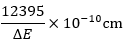•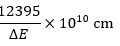SolutionQ8.Which of the following nuclei is unstable?>
••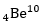••Solution
Part

Q9.Thiosulphate ion, S2O3^(2-) on acidification changes to SO_2 along with precipitation of sulphur
^35 S^32 SO_3^(2-)+2H^+→H_2 O+SO_2+S
Which is the correct statement?
•   ^35 S is in sulphur
•   ^35 Sis in SO_2
•   ^35 Sis in both
•   ^35 Sis in none
Solution
Part

Q10. The electrons, identified by quantum numbers n and l
n=4,l=1
n=4,l=0
n=3,l=2
n=3,l=1
Can be placed in the order of increasing energy, from the lowest to highest, as
•  iv
•  ii
•  i
• iii
Solution#### Written by: AUTHORNAME

AUTHORDESCRIPTION## Want to know more

Please fill in the details below:

## Latest NEET Articles\$type=three\$c=3\$author=hide\$comment=hide\$rm=hide\$date=hide\$snippet=hide

Name

ltr
item
BEST NEET COACHING CENTER | BEST IIT JEE COACHING INSTITUTE | BEST NEET & IIT JEE COACHING: STRUCTURE OF ATOM Quiz-1
STRUCTURE OF ATOM Quiz-1